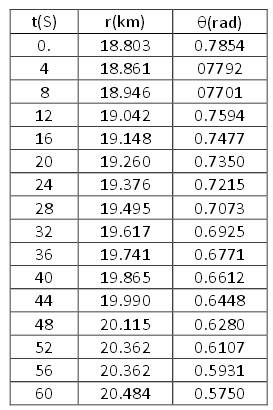# Magnitudes of Velocity and Acceleration

• Tom Lor

## Homework Statement

I need to determine the magnitude of velocity and acceleration for an aircraft moving away from a fixed position (for example a radar station). I need to calculate the magnitude of velocity and acceleration for each time step.

The following table of information is given## Homework Equations

The magnitude of velocity is given as
$$\sqrt{(\frac{dr}{dt})^2+(r\frac{d\theta}{dt})^2}$$

And acceleration
$$[\frac{d^2r}{dt^2}-r(\frac{d\theta}{dt})^2]^2+[r\frac{d^2\theta}{dt^2}+2\frac{dr}{dt}\frac{d\theta}{dt}]^2$$

3.0 Attempt
I am currently in the process of programming this into FORTRAN to get results but I am struggling to work out how to calculate. I am just hoping someone can point me in the right direction. I know I need to calculate the first and second derivatives but the fact I need to take an angle into account is confusing me somewhat. Also to add, how can I calculate a magnitude of velocity for time 0?

I am currently in the process of programming this into FORTRAN to get results but I am struggling to work out how to calculate. I am just hoping someone can point me in the right direction.
The best is to use the finite difference method.

Also to add, how can I calculate a magnitude of velocity for time 0?
There are different approaches you can take. The simplest is to used a forward difference, which uses only information at the current and later times.

Thanks for that, I will try and implement into my program!